# Using the ‘BayesFactor’ package, version 0.9.2+

#### 21 September, 2023BayesFactor

Stable version: CRAN page - Package NEWS (including version changes)

Development version: Development page - Development package NEWS

### Introduction

The BayesFactor package enables the computation of Bayes factors in standard designs, such as one- and two- sample designs, ANOVA designs, and regression. The Bayes factors are based on work spread across several papers. This document is designed to show users how to compute Bayes factors using the package by example. It is not designed to present the models used in the comparisons in detail; for that, see the BayesFactor help and especially the references listed in this manual. Complete references are given at the end of this document.

If you need help or think you’ve found a bug, please use the links at the top of this document to contact the developers. When asking a question or reporting a bug, please send example code and data, the exact errors you’re seeing (a cut-and-paste from the R console will work) and instructions for reproducing it. Also, report the output of BFInfo() and sessionInfo(), and let us know what operating system you’re running.

The BayesFactor package must be installed and loaded before it can be used. Installing the package can be done in several ways and will not be covered here. Once it is installed, use the library function to load it:

library(BayesFactor)

This command will make the BayesFactor package ready to use.

### Some useful functions

The table below lists some of the functions in the BayesFactor package that will be demonstrated in this manual. For more complete help on the use of these functions, see the corresponding help() page in R.

Function Description
ttestBF Bayes factors for one- and two- sample designs
anovaBF Bayes factors comparing many ANOVA models
regressionBF Bayes factors comparing many linear regression models
generalTestBF Bayes factors for all restrictions on a full model (0.9.4+)
lmBF Bayes factors for specific linear models (ANOVA or regression)
correlationBF Bayes factors for linear correlations
proportionBF Bayes factors for tests of single proportions
contingencyTableBF Bayes factors for contingency tables
posterior Sample from the posterior distribution of the numerator of a Bayes factor object
recompute Recompute a Bayes factor or MCMC chain, possibly increasing the precision of the estimate
compare Compare two models; typically used to compare two models in BayesFactor MCMC objects

#### Functions to manipulate Bayes factor objects

The t test section below has examples showing how to manipulate Bayes factor objects, but all these functions will work with Bayes factors generated from any function in the BayesFactor package.

Function Description
/ Divide two Bayes factor objects to create new model comparisons, or invert with 1/
t “Flip” (transpose) a Bayes factor object
c Concatenate two Bayes factor objects together, assuming they have the same denominator
[ Use indexing to select a subset of the Bayes factors
plot plot a Bayes factor object
sort Sort a Bayes factor object
is.na Determine whether a Bayes factor object contains missing values
head,tail Return the n highest or lowest Bayes factor in an object
max, min Return the highest or lowest Bayes factor in an object
which.max,which.min Return the index of the highest or lowest Bayes factor
as.vector Convert to a simple vector (denominator will be lost!)
as.data.frame Convert to data.frame (denominator will be lost!)

### One- and two-sample designs (t tests)

The ttestBF function is used to obtain Bayes factors corresponding to tests of a single sample’s mean, or tests that two independent samples have the same mean.

#### One-sample tests (and paired)

We use the sleep data set in R to demonstrate a one-sample t test. This is a paired design; for details about the data set, see ?sleep. One way of analyzing these data is to compute difference scores by subtracting a participant’s score in one condition from their score in the other:

data(sleep)

## Compute difference scores
diffScores = sleep$extra[1:10] - sleep$extra[11:20]

t.test(diffScores)
##
##  One Sample t-test
##
## data:  diffScores
## t = -4, df = 9, p-value = 0.003
## alternative hypothesis: true mean is not equal to 0
## 95 percent confidence interval:
##  -2.46 -0.70
## sample estimates:
## mean of x
##     -1.58

We can do a Bayesian version of this analysis using the ttestBF function, which performs the “JZS” t test described by Rouder, Speckman, Sun, Morey, and Iverson (2009). In this model, the true standardized difference $$\delta=(\mu-\mu_0)/\sigma_\epsilon$$ is assumed to be 0 under the null hypothesis, and $$\text{Cauchy}(\text{scale}=r)$$ under the alternative. The default $$r$$ scale in BayesFactor for t tests is $$\sqrt{2}/2$$. See ?ttestBF for more details.

bf = ttestBF(x = diffScores)
## Equivalently:
## bf = ttestBF(x = sleep$extra[1:10],y=sleep$extra[11:20], paired=TRUE)
bf
## Bayes factor analysis
## --------------
##  Alt., r=0.707 : 17.3 ±0%
##
## Against denominator:
##   Null, mu = 0
## ---
## Bayes factor type: BFoneSample, JZS

The bf object contains the Bayes factor, and shows the numerator and denominator models for the Bayes factor comparison. In our case, the Bayes factor for the comparison of the alternative versus the null is 17.259. After the Bayes factor is a proportional error estimate on the Bayes factor.

There are a number of operations we can perform on our Bayes factor, such as taking the reciprocal:

1 / bf
## Bayes factor analysis
## --------------
##  Null, mu=0 : 0.0579 ±0%
##
## Against denominator:
##   Alternative, r = 0.707106781186548, mu =/= 0
## ---
## Bayes factor type: BFoneSample, JZS

or sampling from the posterior of the numerator model:

chains = posterior(bf, iterations = 1000)
summary(chains)
##
## Iterations = 1:1000
## Thinning interval = 1
## Number of chains = 1
## Sample size per chain = 1000
##
## 1. Empirical mean and standard deviation for each variable,
##    plus standard error of the mean:
##
##        Mean     SD Naive SE Time-series SE
## mu    -1.42  0.436   0.0138         0.0154
## sig2   2.02  1.157   0.0366         0.0395
## delta -1.11  0.427   0.0135         0.0162
## g      6.26 58.623   1.8538         1.8538
##
## 2. Quantiles for each variable:
##
##         2.5%    25%   50%    75%  97.5%
## mu    -2.289 -1.705 -1.43 -1.141 -0.597
## sig2   0.744  1.270  1.69  2.446  5.223
## delta -1.973 -1.383 -1.08 -0.813 -0.347
## g      0.176  0.592  1.13  2.928 33.734

The posterior function returns a object of type BFmcmc, which inherits the methods of the mcmc class from the coda package. We can thus use summary, plot, and other useful methods on the result of posterior. If we were unhappy with the number of iterations we sampled for chains, we can recompute with more iterations, and then plot the results:

chains2 = recompute(chains, iterations = 10000)
plot(chains2[,1:2])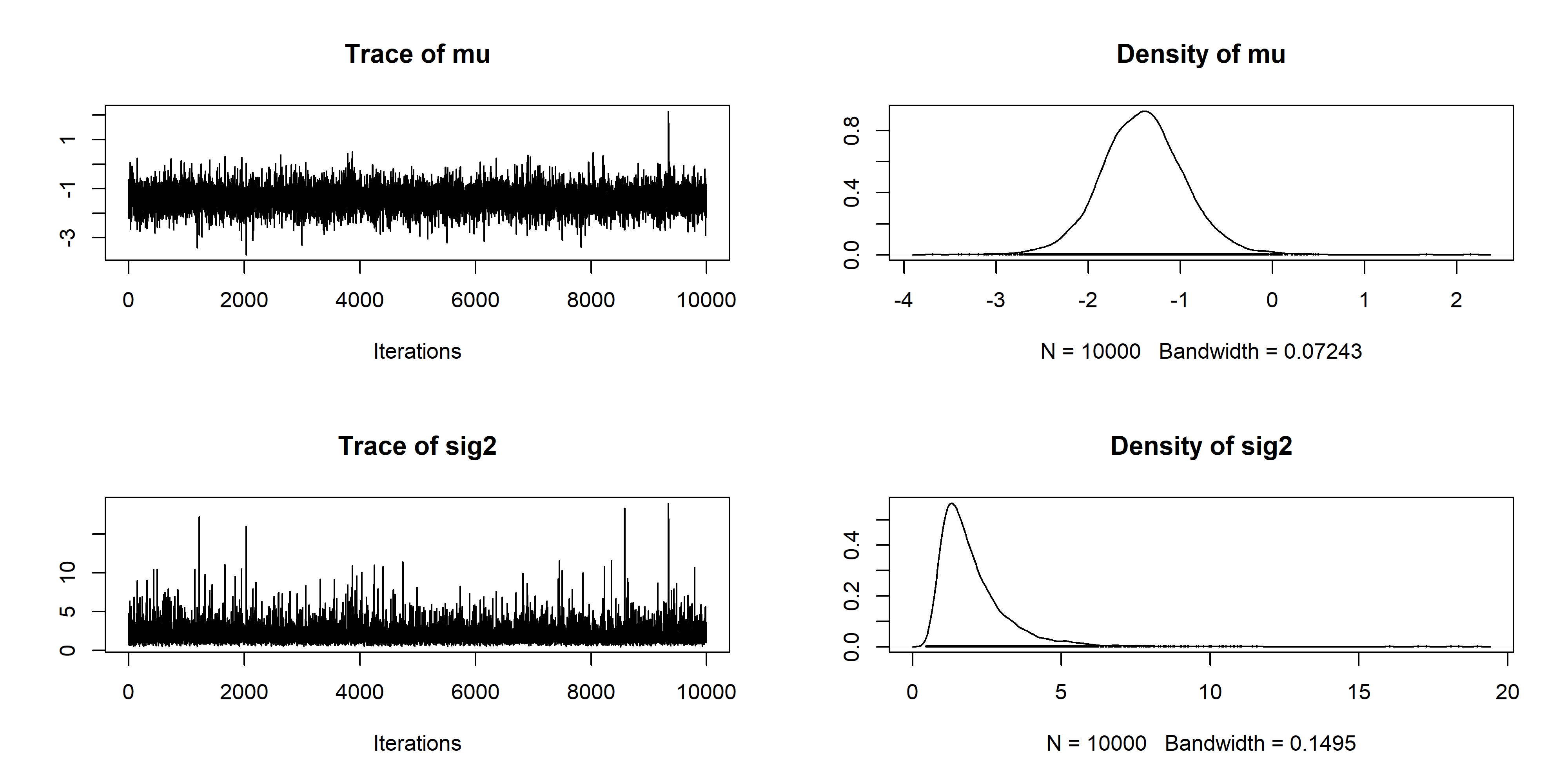Directional hypotheses can also be tested with ttestBF (Morey & Rouder, 2011). The argument nullInterval can be passed as a vector of length 2, and defines an interval to compare to the point null. If null interval is defined, two Bayes factors are returned: the Bayes factor of the null interval against the alternative, and the Bayes factor of the complement of the interval to the point null.

Suppose, for instance, we wanted to test the one-sided hypotheses that $$\delta<0$$ versus the point null. We set nullInterval to c(-Inf,0):

bfInterval = ttestBF(x = diffScores, nullInterval=c(-Inf,0))
bfInterval
## Bayes factor analysis
## --------------
##  Alt., r=0.707 -Inf<d<0    : 34.4  ±0%
##  Alt., r=0.707 !(-Inf<d<0) : 0.101 ±0%
##
## Against denominator:
##   Null, mu = 0
## ---
## Bayes factor type: BFoneSample, JZS

We may not be interested in tests against the point null. If we are interested in the Bayes factor test that $$\delta<0$$ versus $$\delta>0$$ we can compute it using the result above. Since the object contains two Bayes factors, both with the same denominator, and $\left.\frac{A}{C}\middle/\frac{B}{C}\right. = \frac{A}{B},$ we can divide the two Bayes factors in bfInferval to obtain the desired test:

bfInterval / bfInterval
## Bayes factor analysis
## --------------
##  Alt., r=0.707 -Inf<d<0 : 341 ±0%
##
## Against denominator:
##   Alternative, r = 0.707106781186548, mu =/= 0 !(-Inf<d<0)
## ---
## Bayes factor type: BFoneSample, JZS

The Bayes factor is about 340.

When we have multiple Bayes factors that all have the same denominator, we can concatenate them into one object using the c function. Since bf and bfInterval both share the point null denominator, we can do this:

allbf = c(bf, bfInterval)
allbf
## Bayes factor analysis
## --------------
##  Alt., r=0.707             : 17.3  ±0%
##  Alt., r=0.707 -Inf<d<0    : 34.4  ±0%
##  Alt., r=0.707 !(-Inf<d<0) : 0.101 ±0%
##
## Against denominator:
##   Null, mu = 0
## ---
## Bayes factor type: BFoneSample, JZS

The object allbf now contains three Bayes factors, all of which share the same denominator. If you try to concatenate Bayes factors that do not share the same denominator, BayesFactor will return an error.

When you have a Bayes factor object with several numerators, there are several interesting ways to manipulate them. For instance, we can plot the Bayes factor object to obtain a graphical representation of the Bayes factors:

plot(allbf)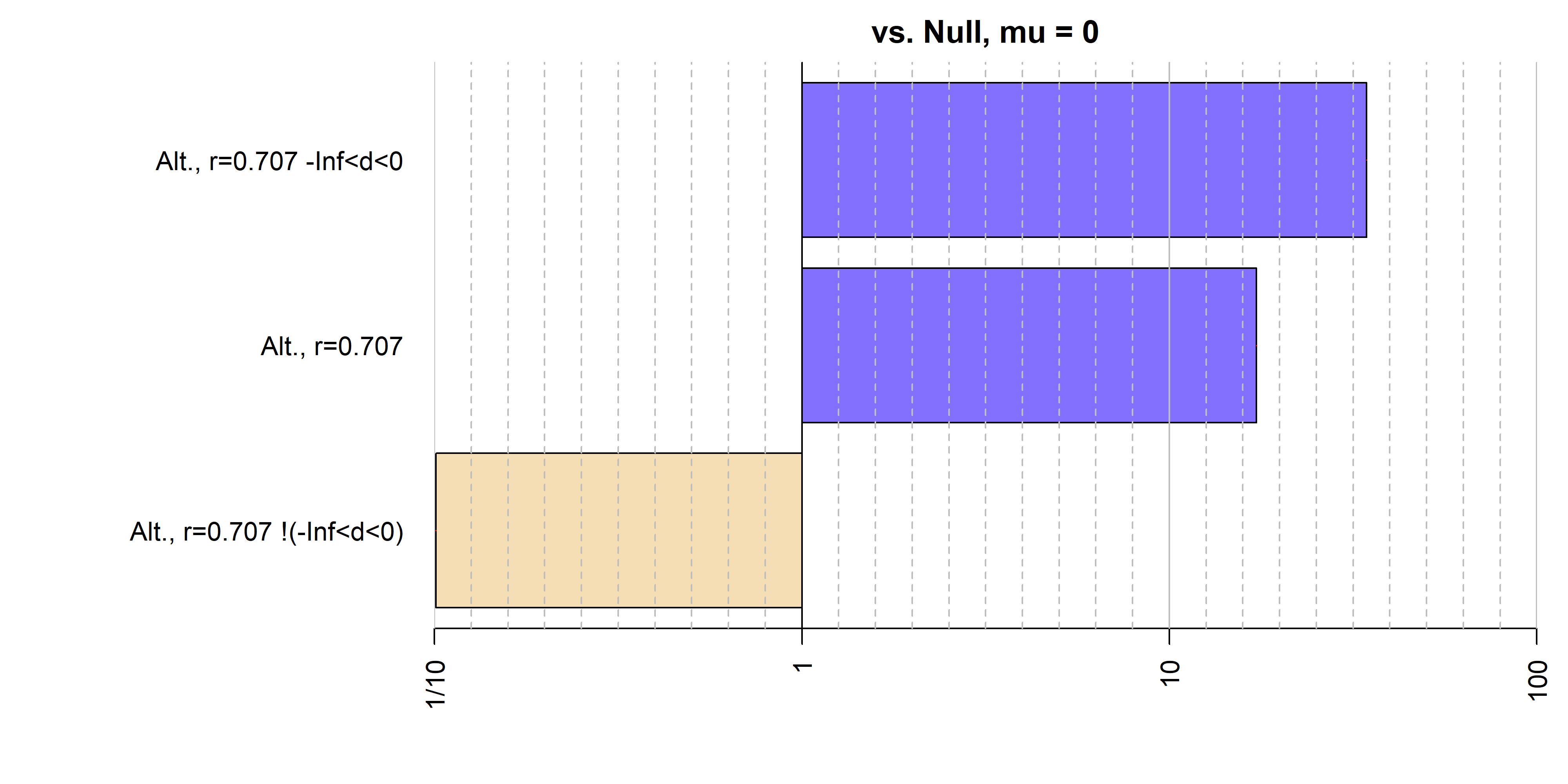We can also divide a Bayes factor object by itself — or by a subset of itself — to obtain pairwise comparisons:

bfmat = allbf / allbf
bfmat
##                            denominator
## numerator                   Alt., r=0.707 Alt., r=0.707 -Inf<d<0
##   Alt., r=0.707                   1.00000                0.50146
##   Alt., r=0.707 -Inf<d<0          1.99416                1.00000
##   Alt., r=0.707 !(-Inf<d<0)       0.00584                0.00293
##                            denominator
## numerator                   Alt., r=0.707 !(-Inf<d<0)
##   Alt., r=0.707                                   171
##   Alt., r=0.707 -Inf<d<0                          341
##   Alt., r=0.707 !(-Inf<d<0)                         1

The resulting object is of type BFBayesFactorList, and is a list of Bayes factor comparisons all of the same numerators compared to different denominators. The resulting matrix can be subsetted to return individual Bayes factor objects, or new BFBayesFactorLists:

bfmat[,2]
## Bayes factor analysis
## --------------
##  Alt., r=0.707             : 0.501   ±0%
##  Alt., r=0.707 -Inf<d<0    : 1       ±0%
##  Alt., r=0.707 !(-Inf<d<0) : 0.00293 ±0%
##
## Against denominator:
##   Alternative, r = 0.707106781186548, mu =/= 0 -Inf<d<0
## ---
## Bayes factor type: BFoneSample, JZS
bfmat[1,]
##                denominator
## numerator       Alt., r=0.707 Alt., r=0.707 -Inf<d<0 Alt., r=0.707 !(-Inf<d<0)
##   Alt., r=0.707             1                  0.501                       171

and they can also be transposed:

bfmat[,1:2]
##                            denominator
## numerator                   Alt., r=0.707 Alt., r=0.707 -Inf<d<0
##   Alt., r=0.707                   1.00000                0.50146
##   Alt., r=0.707 -Inf<d<0          1.99416                1.00000
##   Alt., r=0.707 !(-Inf<d<0)       0.00584                0.00293
t(bfmat[,1:2])
##                         denominator
## numerator                Alt., r=0.707 Alt., r=0.707 -Inf<d<0
##   Alt., r=0.707                   1.00                  0.501
##   Alt., r=0.707 -Inf<d<0          1.99                  1.000
##                         denominator
## numerator                Alt., r=0.707 !(-Inf<d<0)
##   Alt., r=0.707                                171
##   Alt., r=0.707 -Inf<d<0                       341

If these values are desired in matrix form, the as.matrix function can be used to obtain a matrix.

#### Two-sample test (independent groups)

The ttestBF function can also be used to compute Bayes factors in the two sample case as well. We use the chickwts data set to demonstrate the two-sample t test. The chickwts data set has six groups, but we reduce it to two for the demonstration.

data(chickwts)

## Restrict to two groups
chickwts = chickwts[chickwts$feed %in% c("horsebean","linseed"),] ## Drop unused factor levels chickwts$feed = factor(chickwts$feed) ## Plot data plot(weight ~ feed, data = chickwts, main = "Chick weights")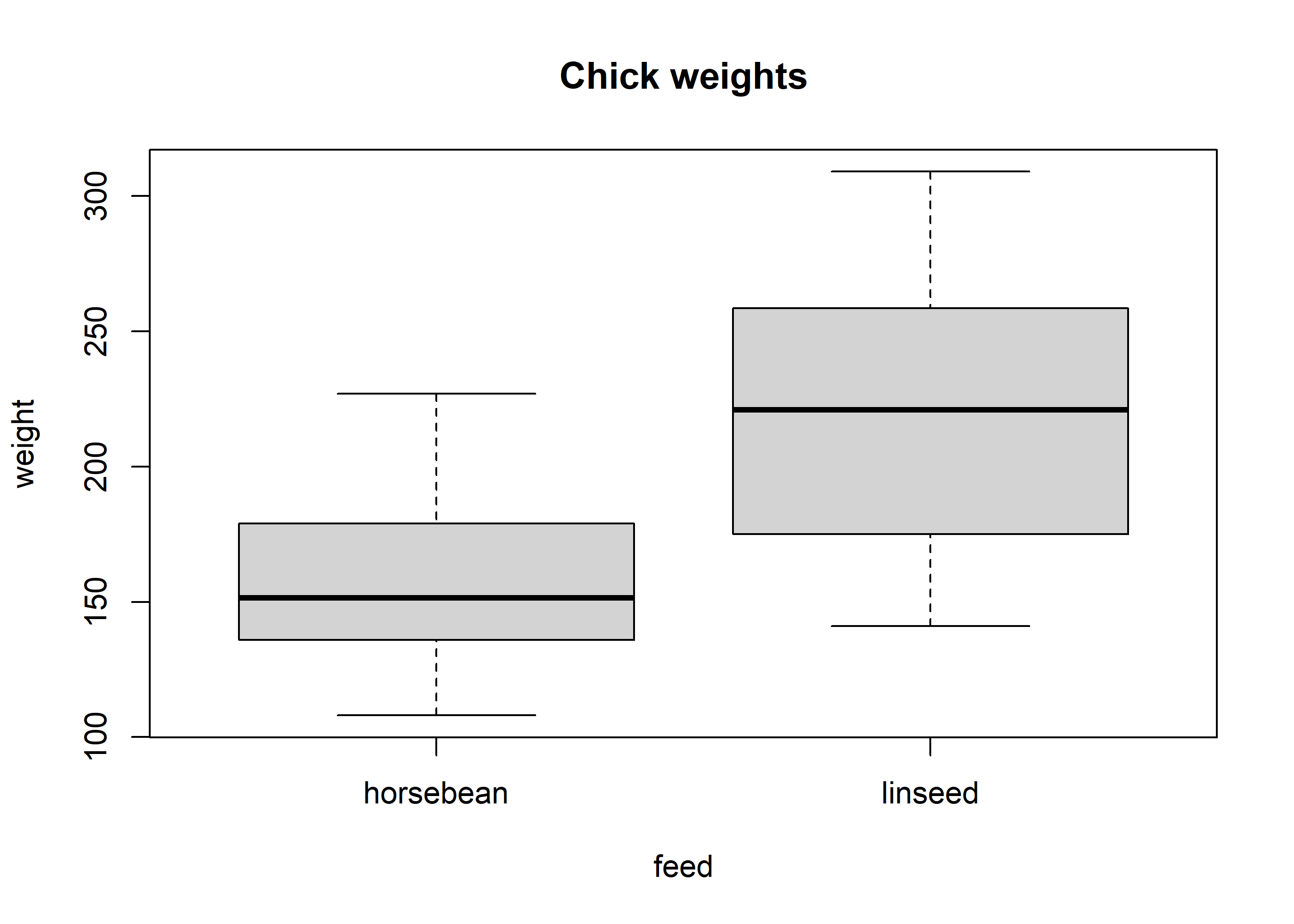Chick weight appears to be affected by the feed type. ## traditional t test t.test(weight ~ feed, data = chickwts, var.eq=TRUE) ## ## Two Sample t-test ## ## data: weight by feed ## t = -3, df = 20, p-value = 0.008 ## alternative hypothesis: true difference in means between group horsebean and group linseed is not equal to 0 ## 95 percent confidence interval: ## -100.2 -16.9 ## sample estimates: ## mean in group horsebean mean in group linseed ## 160 219 We can also compute the corresponding Bayes factor. There are two ways of specifying a two-sample test: the formula interface and through the x and y arguments. We show the formula interface here: ## Compute Bayes factor bf = ttestBF(formula = weight ~ feed, data = chickwts) bf ## Bayes factor analysis ## -------------- ##  Alt., r=0.707 : 5.98 ±0% ## ## Against denominator: ## Null, mu1-mu2 = 0 ## --- ## Bayes factor type: BFindepSample, JZS As before, we can sample from the posterior distribution for the numerator model: chains = posterior(bf, iterations = 10000) plot(chains[,2])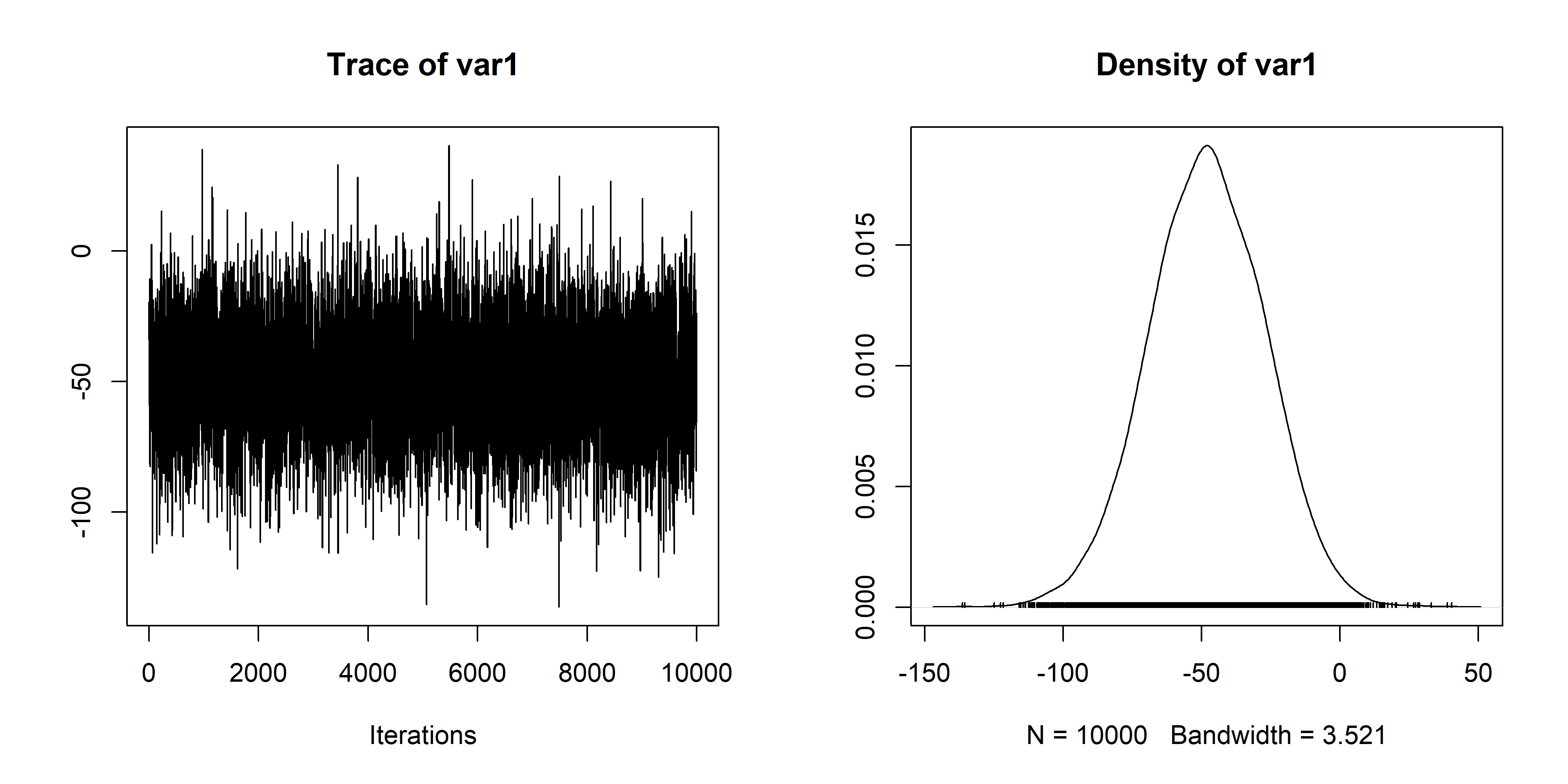Note that the samples assume an (equivalent) ANOVA model; see ?ttestBF and for notes on the differences in interpretation of the $$r$$ scale parameter between the two models. ### Meta-analytic t tests (0.9.8+) Rouder and Morey (2011; link) discuss a meta-analytic extension of the $$t$$ test, whereby multiple $$t$$ statistics, along with their corresponding sample sizes, are combined in a single meta-analytic analysis. The $$t$$ statistics are assumed to arise from a a common effect size $$\delta$$. The prior for the effect size $$\delta$$ is the same as that for the $$t$$ tests described above. The meta.ttestBF function is used to perform meta-analytic $$t$$ tests. It requires as input a vector of $$t$$ statistics, and one or two vectors of sample sizes (arguments n1 and n2). For a set of one-sample $$t$$ statistics, n1 should be provided; for two-sample analyses, both n1 and n2 should be provided. As an example, we will replicate the analysis of Rouder & Morey (2011), using $$t$$ statistics from Bem (2010; see Rouder & Morey for reference). We begin by defining the one-sample $$t$$ statistics and sample sizes: ## Bem's t statistics from four selected experiments t = c(-.15, 2.39, 2.42, 2.43) N = c(100, 150, 97, 99) Rouder and Morey opted for a one-sided analysis, and used an $$r$$ scale parameter of 1 (instead of the current default in BayesFactor of $$\sqrt{2}/2$$). bf = meta.ttestBF(t=t, n1=N, nullInterval=c(0,Inf), rscale=1) bf ## Bayes factor analysis ## -------------- ##  Alt., r=1 0<d<Inf : 38.7 ±0% ##  Alt., r=1 !(0<d<Inf) : 0.00803 ±0% ## ## Against denominator: ## Null, d = 0 ## --- ## Bayes factor type: BFmetat, JZS Notice that as above, the analysis yields a Bayes factor for our selected interval against the null, as well as the Bayes factor for the complement of the interval against the null. We can also sample from the posterior distribution of the standardized effect size $$\delta$$, as above, using the posterior function: ## Do analysis again, without nullInterval restriction bf = meta.ttestBF(t=t, n1=N, rscale=1) ## Obtain posterior samples chains = posterior(bf, iterations = 10000) ## Independent-candidate M-H acceptance rate: 98% plot(chains)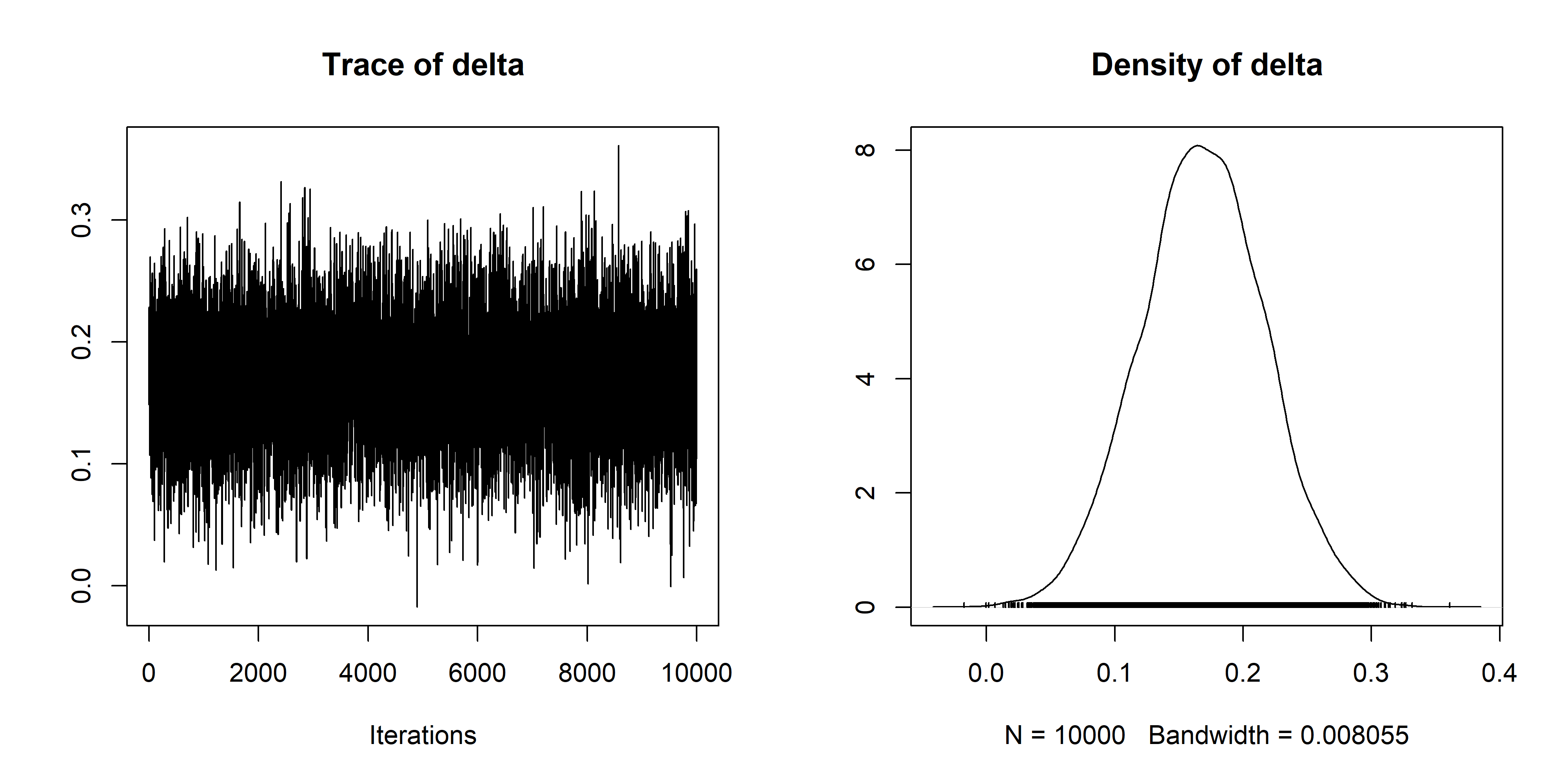Notice that the posterior samples will respect the nullInterval argument if given; in order to get unrestricted samples, perform an analysis with no interval restriction and pass it to the posterior function. See ?meta.ttestBF for more information. ### ANOVA The BayesFactor package has two main functions that allow the comparison of models with factors as predictors (ANOVA): anovaBF, which computes several model estimates at once, and lmBF, which computes one comparison at a time. We begin by demonstrating a 3x2 fixed-effect ANOVA using the ToothGrowth data set. For details about the data set, see ?ToothGrowth. #### Fixed-effects ANOVA The ToothGrowth data set contains three columns: len, the dependent variable, each of which is the length of a guinea pig’s tooth after treatment with Vitamin C; supp, which is the supplement type (orange juice or ascorbic acid); and dose, which is the amount of Vitamin C administered. data(ToothGrowth) ## Example plot from ?ToothGrowth coplot(len ~ dose | supp, data = ToothGrowth, panel = panel.smooth, xlab = "ToothGrowth data: length vs dose, given type of supplement")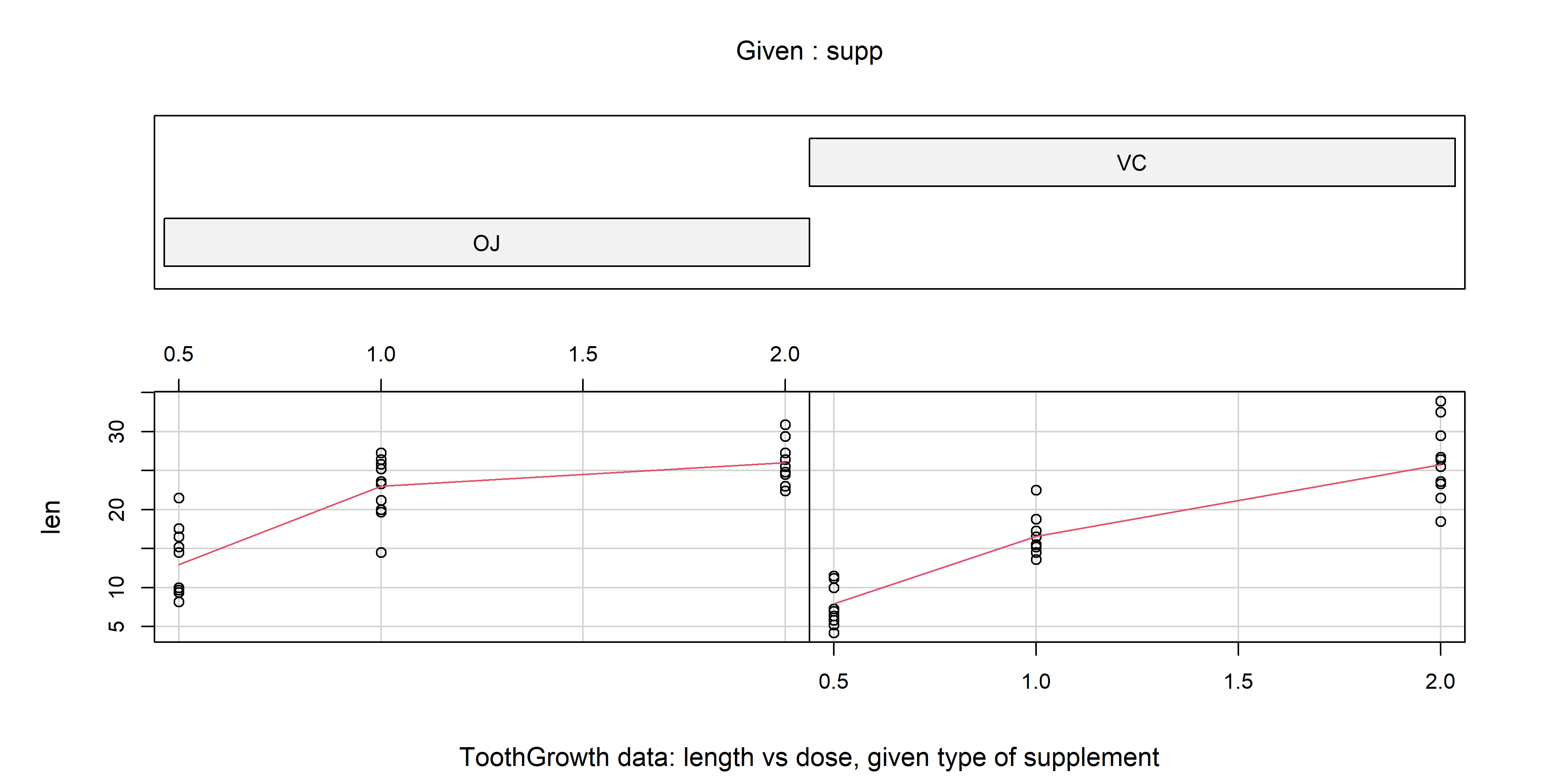## Treat dose as a factor ToothGrowth$dose = factor(ToothGrowth$dose) levels(ToothGrowth$dose) = c("Low", "Medium", "High")

summary(aov(len ~ supp*dose, data=ToothGrowth))
##             Df Sum Sq Mean Sq F value  Pr(>F)
## supp         1    205     205   15.57 0.00023 ***
## dose         2   2426    1213   92.00 < 2e-16 ***
## supp:dose    2    108      54    4.11 0.02186 *
## Residuals   54    712      13
## ---
## Signif. codes:  0 '***' 0.001 '**' 0.01 '*' 0.05 '.' 0.1 ' ' 1

There appears to be a large effect of the dosage, a small effect of the supplement type, and perhaps a hint of an interaction. The anovaBF function will compute the Bayes factors of all models against the intercept-only model; by default, it will choose the subset of all models in which which an interaction can only be included if all constituent effects or interactions are included (argument whichModels is set to withmain, indicating that interactions can only enter in with their main effects). However, this setting can be changed, as we will demonstrate. First, we show the default behavior.

bf = anovaBF(len ~ supp*dose, data=ToothGrowth)
bf
## Bayes factor analysis
## --------------
##  supp                    : 1.2      ±0.01%
##  dose                    : 4.98e+12 ±0%
##  supp + dose             : 2.92e+14 ±1.58%
##  supp + dose + supp:dose : 7.44e+14 ±1.01%
##
## Against denominator:
##   Intercept only
## ---
## Bayes factor type: BFlinearModel, JZS

The function will build the requested models from the terms included in the right-hand side of the formula; we could have specified the sum of the two terms, and we would have gotten the same models.

The Bayes factor analysis is consistent with the classical ANOVA analysis; the favored model is the full model, with both main effects and the two-way interaction. Suppose we were interested in comparing the two main-effects model and the full model to the dose-only model. We could use indexing and division, along with the plot function, to see a graphical representation of these comparisons:

plot(bf[3:4] / bf)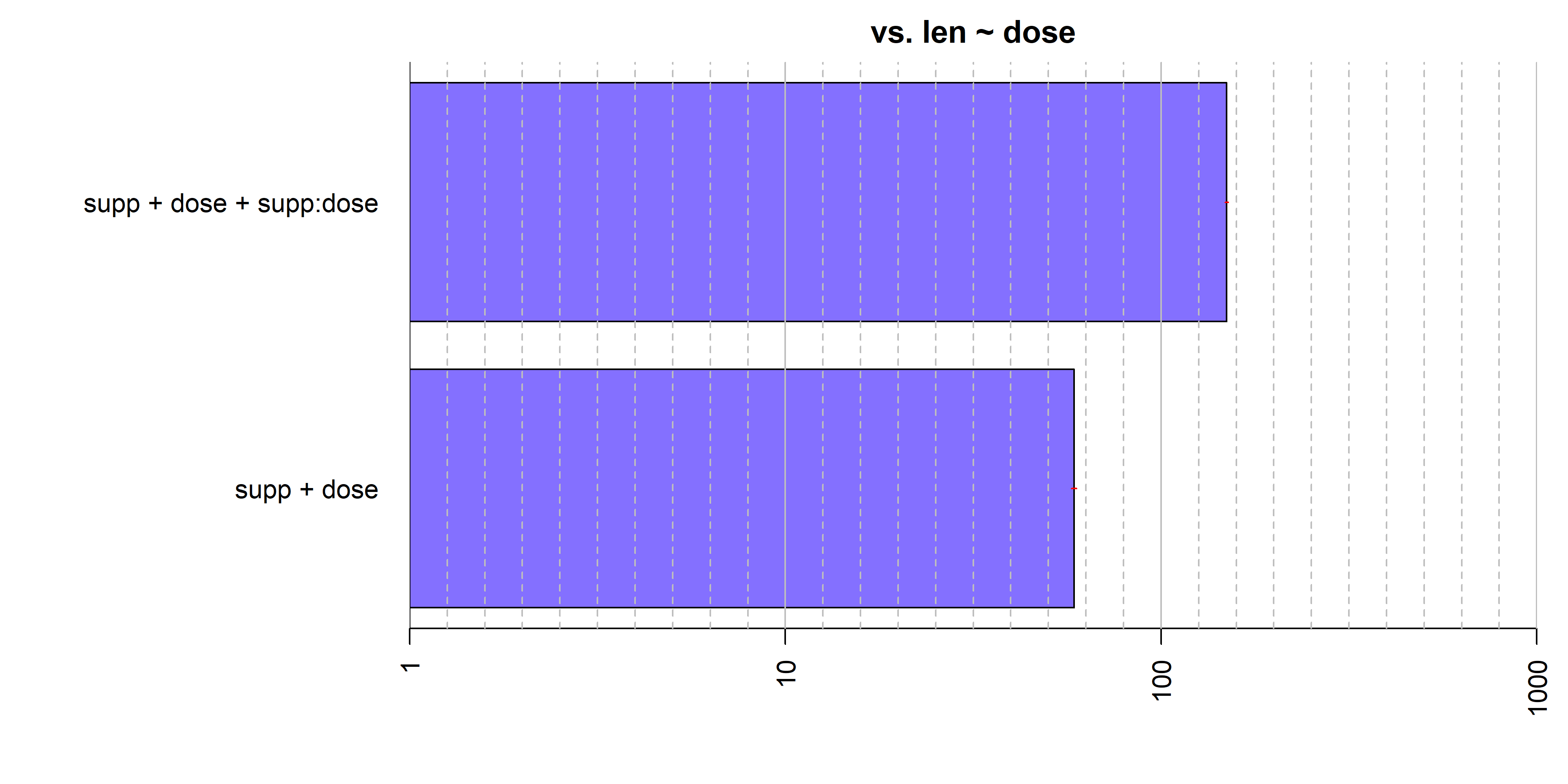The model with the main effect of supp and the supp:dose interaction is preferred quite strongly over the dose-only model.

There are a number of other options for how to select subsets of models to test. The whichModels argument to anovaBF controls which subsets are tested. As described previously, the default is withmain, where interactions are only allowed if all constituent sub-effects are included. The other three options currently available are all, which tests all models; top, which includes the full model and all models that can be formed by removing one interaction or main effect; and bottom, which adds single effects one at a time to the null model.

The argument whichModels='all' should be used with caution: a three-way ANOVA model will contain $$2^{2^3-1}-1 = 127$$ model comparisons; a four-way ANOVA, $$2^{2^4-1}-1 = 32767$$ models, and a five-way ANOVA just over 2.1 billion models. Depending on the speed of your computer, a four-way ANOVA may take several hours to a day, but a five-way ANOVA is probably not feasible.

One alternative is whichModels='top', which reduces the number of comparisons to $$2^k-1$$, where $$k$$ is the number of factors, which is manageable. In orthogonal designs, one can construct tests of each main effect or interaction by comparing the full model to the model with all effects except the one of interest:

bf = anovaBF(len ~ supp*dose, data=ToothGrowth, whichModels="top")
bf
## Bayes factor top-down analysis
## --------------
## When effect is omitted from supp + dose + supp:dose , BF is...
##  Omit dose:supp : 0.385    ±3.32%
##  Omit dose      : 7.11e-16 ±12.2%
##  Omit supp      : 0.011    ±4.17%
##
## Against denominator:
##   len ~ supp + dose + supp:dose
## ---
## Bayes factor type: BFlinearModel, JZS

Note that all of the Bayes factors are less than 1, indicating that removing any effect from the full model is deleterious.

Another way we can reduce the number of models tested is simply to test only specific models of interest. In the example above, for instance, we might want to compare the model with the interaction to the model with only the main effects, if our effect of interest was the interaction. We can do this with the lmBF function.

bfMainEffects = lmBF(len ~ supp + dose, data = ToothGrowth)
bfInteraction = lmBF(len ~ supp + dose + supp:dose, data = ToothGrowth)
## Compare the two models
bf = bfInteraction / bfMainEffects
bf
## Bayes factor analysis
## --------------
##  supp + dose + supp:dose : 2.79 ±2.51%
##
## Against denominator:
##   len ~ supp + dose
## ---
## Bayes factor type: BFlinearModel, JZS

The model with the interaction effect is preferred by a factor of about 3.

Suppose that we were unhappy with the ~2.5% proportional error on the Bayes factor bf. anovaBF and lmBF use Monte Carlo integration to estimate the Bayes factors. The default number of Monte Carlo samples is 10,000 but this can be increased. We could use the recompute to reduce the error. The recompute function performs the sampling required to build the Bayes factor object again:

newbf = recompute(bf, iterations = 500000)
newbf
## Bayes factor analysis
## --------------
##  supp + dose + supp:dose : 2.74 ±0.51%
##
## Against denominator:
##   len ~ supp + dose
## ---
## Bayes factor type: BFlinearModel, JZS

The proportional error is now below 1%.

As before, we can use MCMC methods to estimate parameters through the posterior function:

## Sample from the posterior of the full model
chains = posterior(bfInteraction, iterations = 10000)
## 1:13 are the only "interesting" parameters
summary(chains[,1:13])
##
## Iterations = 1:10000
## Thinning interval = 1
## Number of chains = 1
## Sample size per chain = 10000
##
## 1. Empirical mean and standard deviation for each variable,
##    plus standard error of the mean:
##
##                         Mean    SD Naive SE Time-series SE
## mu                    18.819 0.487  0.00487        0.00487
## supp-OJ                1.679 0.488  0.00488        0.00549
## supp-VC               -1.679 0.488  0.00488        0.00549
## dose-Low              -8.069 0.683  0.00683        0.00705
## dose-Medium            0.910 0.680  0.00680        0.00666
## dose-High              7.159 0.684  0.00684        0.00710
## supp:dose-OJ.&.Low     0.562 0.603  0.00603        0.00616
## supp:dose-OJ.&.Medium  0.822 0.621  0.00621        0.00723
## supp:dose-OJ.&.High   -1.384 0.663  0.00663        0.00833
## supp:dose-VC.&.Low    -0.562 0.603  0.00603        0.00616
## supp:dose-VC.&.Medium -0.822 0.621  0.00621        0.00723
## supp:dose-VC.&.High    1.384 0.663  0.00663        0.00833
## sig2                  14.039 2.772  0.02772        0.03260
##
## 2. Quantiles for each variable:
##
##                         2.5%    25%    50%    75%  97.5%
## mu                    17.880 18.492 18.817 19.142 19.765
## supp-OJ                0.719  1.356  1.679  2.002  2.647
## supp-VC               -2.647 -2.002 -1.679 -1.356 -0.719
## dose-Low              -9.421 -8.516 -8.073 -7.608 -6.746
## dose-Medium           -0.418  0.457  0.904  1.370  2.234
## dose-High              5.808  6.696  7.167  7.615  8.516
## supp:dose-OJ.&.Low    -0.613  0.160  0.552  0.945  1.766
## supp:dose-OJ.&.Medium -0.354  0.404  0.801  1.223  2.093
## supp:dose-OJ.&.High   -2.740 -1.825 -1.365 -0.920 -0.154
## supp:dose-VC.&.Low    -1.766 -0.945 -0.552 -0.160  0.613
## supp:dose-VC.&.Medium -2.093 -1.223 -0.801 -0.404  0.354
## supp:dose-VC.&.High    0.154  0.920  1.365  1.825  2.740
## sig2                   9.625 12.062 13.692 15.615 20.373

And we can plot the posteriors of some selected effects:

plot(chains[,4:6])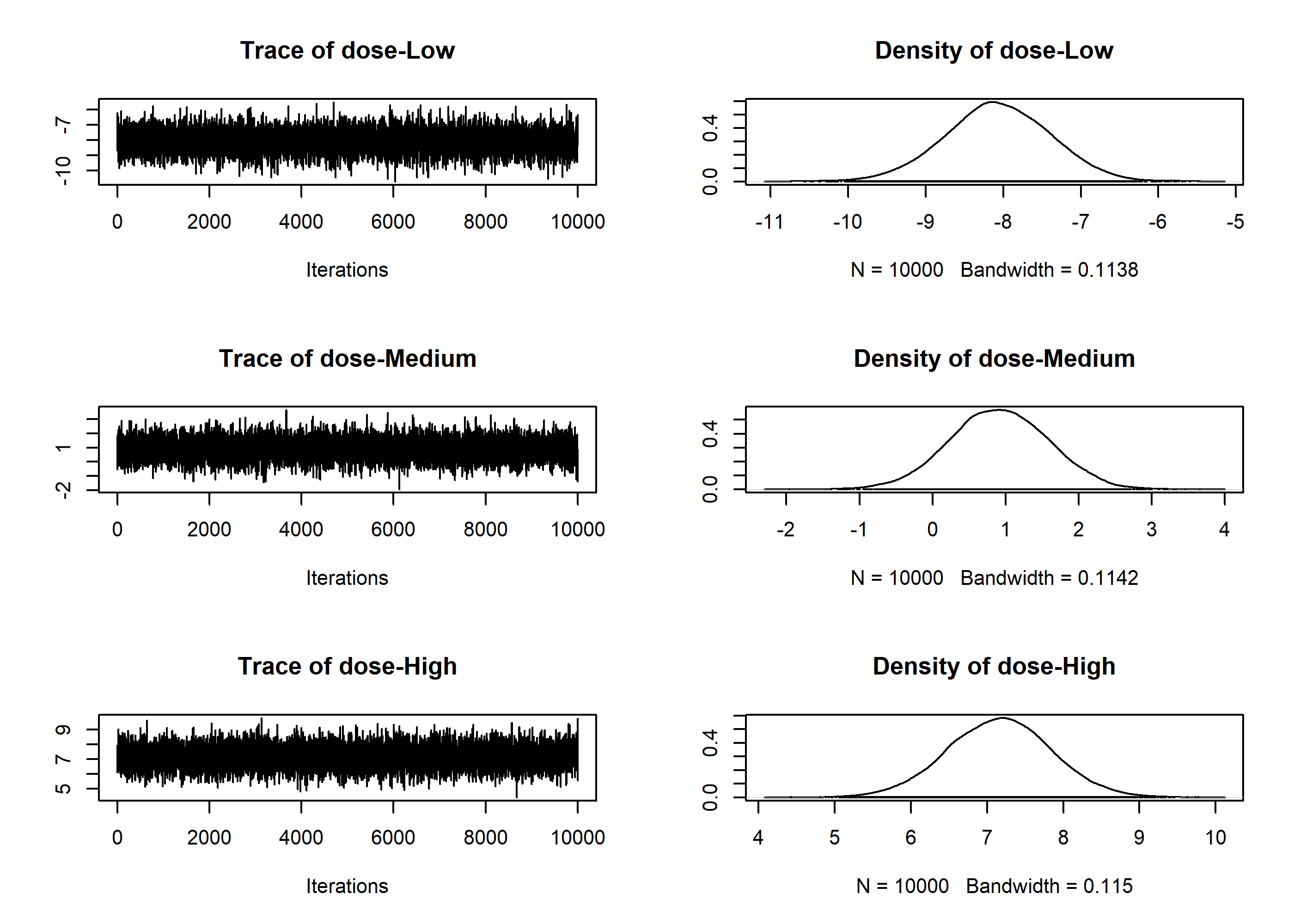#### Mixed models (including repeated measures)

In order to demonstrate the analysis of mixed models using BayesFactor, we will load the puzzles data set, which is part of the BayesFactor package. See ?puzzles for details. The data set consists of four columns: RT the dependent variable, which is the number of seconds that it took to complete a puzzle; ID which is a participant identifier; and shape and color, which are two factors that describe the type of puzzle solved. shape and color each have two levels, and each of 12 participants completed puzzles within combination of shape and color. The design is thus 2x2 factorial within-subjects.

data(puzzles)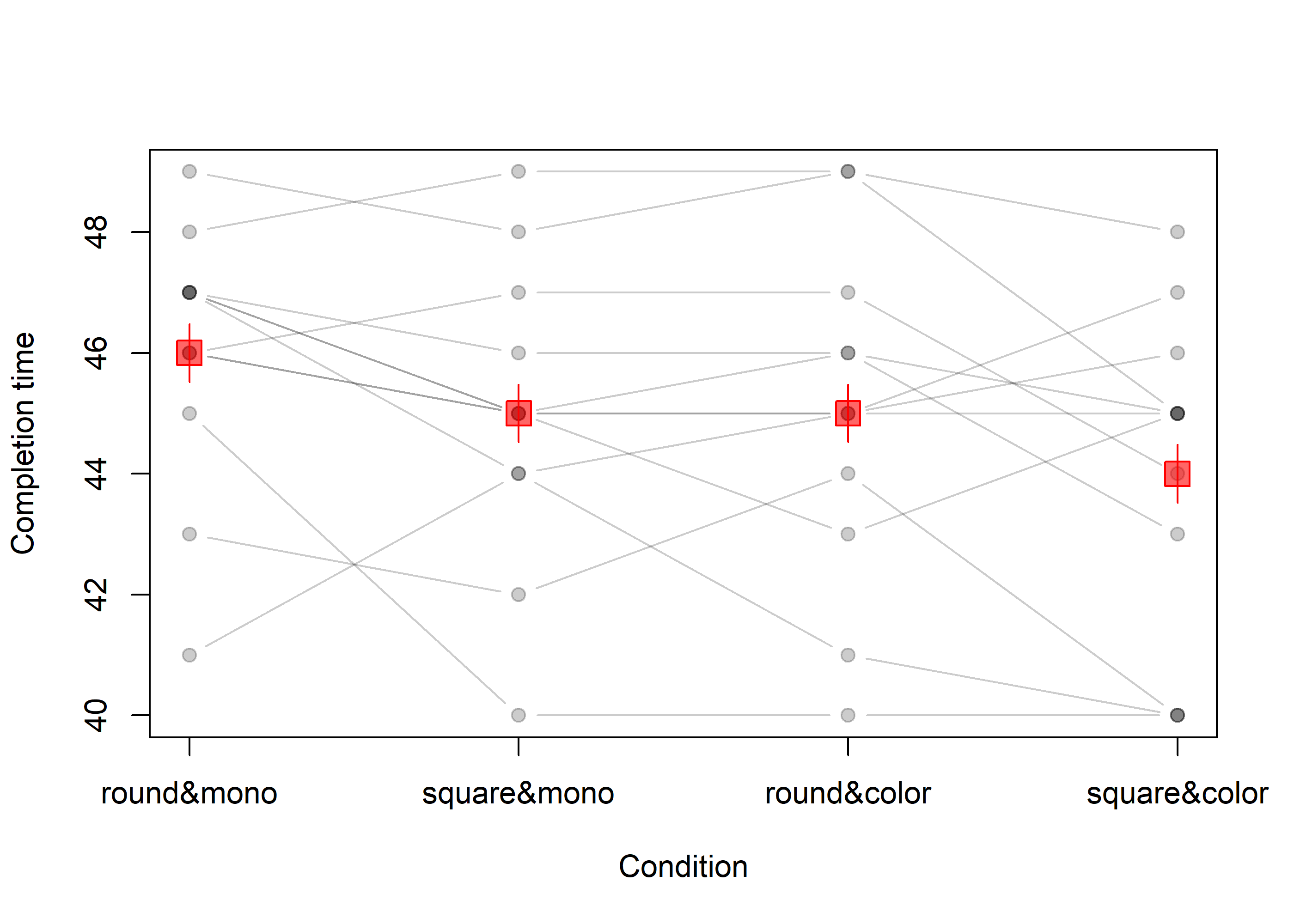(Code for plot omitted) Individual circles joined by lines show participants; red squares/lines show the means and within-subject standard errors. From the plot, there appear to be main effects of color and shape, but no interaction.

summary(aov(RT ~ shape*color + Error(ID/(shape*color)), data=puzzles))
##
## Error: ID
##           Df Sum Sq Mean Sq F value Pr(>F)
## Residuals 11    226    20.6
##
## Error: ID:shape
##           Df Sum Sq Mean Sq F value Pr(>F)
## shape      1   12.0   12.00    7.54  0.019 *
## Residuals 11   17.5    1.59
## ---
## Signif. codes:  0 '***' 0.001 '**' 0.01 '*' 0.05 '.' 0.1 ' ' 1
##
## Error: ID:color
##           Df Sum Sq Mean Sq F value Pr(>F)
## color      1   12.0   12.00    13.9 0.0033 **
## Residuals 11    9.5    0.86
## ---
## Signif. codes:  0 '***' 0.001 '**' 0.01 '*' 0.05 '.' 0.1 ' ' 1
##
## Error: ID:shape:color
##             Df Sum Sq Mean Sq F value Pr(>F)
## shape:color  1    0.0    0.00       0      1
## Residuals   11   30.5    2.77

The classical ANOVA appears to corroborate the impression from the plot. In order to compute the Bayes factor, we must tell anovaBF that ID is an additive effect on top of the other effects (as is typically assumed) and is a random factor. The anovaBF call below shows how this is done:

bf = anovaBF(RT ~ shape*color + ID, data = puzzles,
whichRandom="ID")

We alert anovaBF to the random factor using the whichRandom argument. whichRandom should contain a character vector with the names of all random factors in it. All other factors are assumed to be fixed. The anovaBF will find all the fixed effects in the formula, and compute the Bayes factor for the subset of combinations determined by the whichModels argument (see the previous section). Note that anovaBF does not test random factors; they are assumed to be nuisance factors. The null model in a test with random factors is not the intercept-only model; it is the model containing the random effects. The Bayes factor object bf thus now contains Bayes factors comparing various combinations of the fixed effects and an additive effect of ID against a denominator containing only ID:

bf
## Bayes factor analysis
## --------------
##  shape + ID                       : 2.81 ±0.91%
##  color + ID                       : 2.81 ±0.83%
##  shape + color + ID               : 11.9 ±3%
##  shape + color + shape:color + ID : 4.23 ±2.2%
##
## Against denominator:
##   RT ~ ID
## ---
## Bayes factor type: BFlinearModel, JZS

The main effects model is preferred against all models. We can plot the Bayes factor object to obtain a graphical representation of the model comparisons:

plot(bf)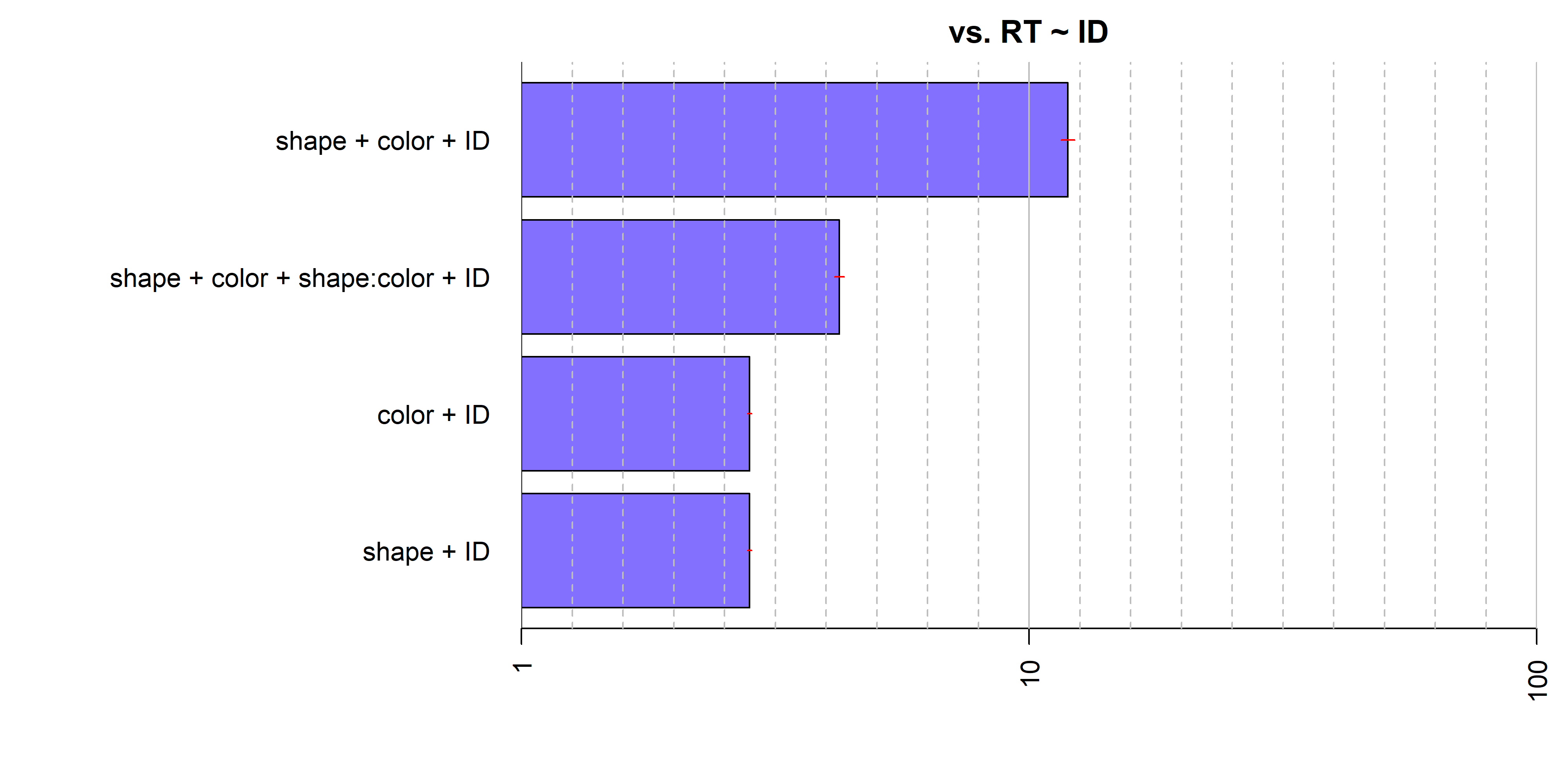Because the anovaBF function does not test random factors, we must use lmBF to build such tests. Doing so is straightforward. Suppose that we wished to test the random effect ID in the puzzles example. We might compare the full model shape + color + shape:color + ID to the same model without ID:

bfWithoutID = lmBF(RT ~ shape*color, data = puzzles)
bfWithoutID
## Bayes factor analysis
## --------------
##  shape * color : 0.143 ±1.14%
##
## Against denominator:
##   Intercept only
## ---
## Bayes factor type: BFlinearModel, JZS

But notice that the denominator model is the intercept-only model; the denominator in the previous analysis was the ID only model. We need to compare the model with no ID effect to the model with only ID:

bfOnlyID = lmBF(RT ~ ID, whichRandom="ID",data = puzzles)
bf2 = bfWithoutID / bfOnlyID
bf2
## Bayes factor analysis
## --------------
##  shape * color : 1.28e-06 ±1.14%
##
## Against denominator:
##   RT ~ ID
## ---
## Bayes factor type: BFlinearModel, JZS

Since our bf object and bf2 object now have the same denominator, we can concatenate them into one Bayes factor object:

bfall = c(bf,bf2)

and we can compare them by dividing:

bf / bf2
## Bayes factor analysis
## --------------
##  shape + color + shape:color + ID : 3307085 ±2.48%
##
## Against denominator:
##   RT ~ shape * color
## ---
## Bayes factor type: BFlinearModel, JZS

The model with ID is preferred by a factor of over 1 million, which is not surprising.

Any model that is a combination of fixed and random factors, including interations between fixed and random factors, can be constructed and tested with lmBF. anovaBF is designed to be a convenience function as is therefore somewhat limited in flexibility with respect to the models types it can test; however, because random effects are often nuisance effects, we believe anovaBF will be sufficient for most researchers’ use.

### Linear regression

Model comparison in multiple linear regression using BayesFactor is done via the approach of Liang, Paulo, Molina, Clyde, and Berger (2008). Further discussion can be found in Rouder & Morey (in press). To demonstrate Bayes factor model comparison in a linear regression context, we use the attitude data set in R. See ?attitude. The attitude consists of the dependent variable rating, along with 6 predictors. We can use BayesFactor to compute the Bayes factors for many models simultaneously, or single Bayes factors against the model containing no predictors.

data(attitude)

lmObj = lm(rating ~ ., data = attitude)
summary(lmObj)
##
## Call:
## lm(formula = rating ~ ., data = attitude)
##
## Residuals:
##     Min      1Q  Median      3Q     Max
## -10.942  -4.356   0.316   5.543  11.599
##
## Coefficients:
##             Estimate Std. Error t value Pr(>|t|)
## (Intercept)  10.7871    11.5893    0.93   0.3616
## complaints    0.6132     0.1610    3.81   0.0009 ***
## privileges   -0.0731     0.1357   -0.54   0.5956
## learning      0.3203     0.1685    1.90   0.0699 .
## raises        0.0817     0.2215    0.37   0.7155
## critical      0.0384     0.1470    0.26   0.7963
## advance      -0.2171     0.1782   -1.22   0.2356
## ---
## Signif. codes:  0 '***' 0.001 '**' 0.01 '*' 0.05 '.' 0.1 ' ' 1
##
## Residual standard error: 7.07 on 23 degrees of freedom
## Multiple R-squared:  0.733,  Adjusted R-squared:  0.663
## F-statistic: 10.5 on 6 and 23 DF,  p-value: 1.24e-05

The period (.) is shorthand for all remaining columns, besides rating. The predictors complaints and learning appear most stongly related to the dependent variable, especially complaints. In order to compute the Bayes factors for many model comparisons at onces, we use the regressionBF function. The most obvious set of all model comparisons is all possible additive models, which is returned by default:

bf = regressionBF(rating ~ ., data = attitude)
length(bf)
##  63

The object bf now contains $$2^p-1$$, or 63, model comparisons. Large numbers of comparisons can get unweildy, so we can use the functions built into R to manipulate the Bayes factor object.

## Choose a specific model
bf["privileges + learning + raises + critical + advance"]
## Bayes factor analysis
## --------------
##  privileges + learning + raises + critical + advance : 51 ±0%
##
## Against denominator:
##   Intercept only
## ---
## Bayes factor type: BFlinearModel, JZS
## Best 6 models
head(bf, n=6)
## Bayes factor analysis
## --------------
##  complaints                      : 417939 ±0.01%
##  complaints + learning           : 207272 ±0%
##  complaints + learning + advance : 88042  ±0%
##  complaints + raises             : 77499  ±0%
##  complaints + privileges         : 75015  ±0%
##  complaints + advance            : 72760  ±0%
##
## Against denominator:
##   Intercept only
## ---
## Bayes factor type: BFlinearModel, JZS
## Worst 4 models
tail(bf, n=4)
## Bayes factor analysis
## --------------
##  privileges + critical + advance : 0.645 ±0%
##  critical                        : 0.449 ±0%
##  advance                         : 0.447 ±0%
##  critical + advance              : 0.239 ±0.01%
##
## Against denominator:
##   Intercept only
## ---
## Bayes factor type: BFlinearModel, JZS
## which model index is the best?
which.max(bf)
## complaints
##          1
## Compare the 5 best models to the best
bf2
## Bayes factor analysis
## --------------
##  complaints                      : 1     ±0%
##  complaints + learning           : 0.496 ±0.01%
##  complaints + learning + advance : 0.211 ±0.01%
##  complaints + raises             : 0.185 ±0.01%
##  complaints + privileges         : 0.179 ±0.01%
##  complaints + advance            : 0.174 ±0.01%
##
## Against denominator:
##   rating ~ complaints
## ---
## Bayes factor type: BFlinearModel, JZS
plot(bf2)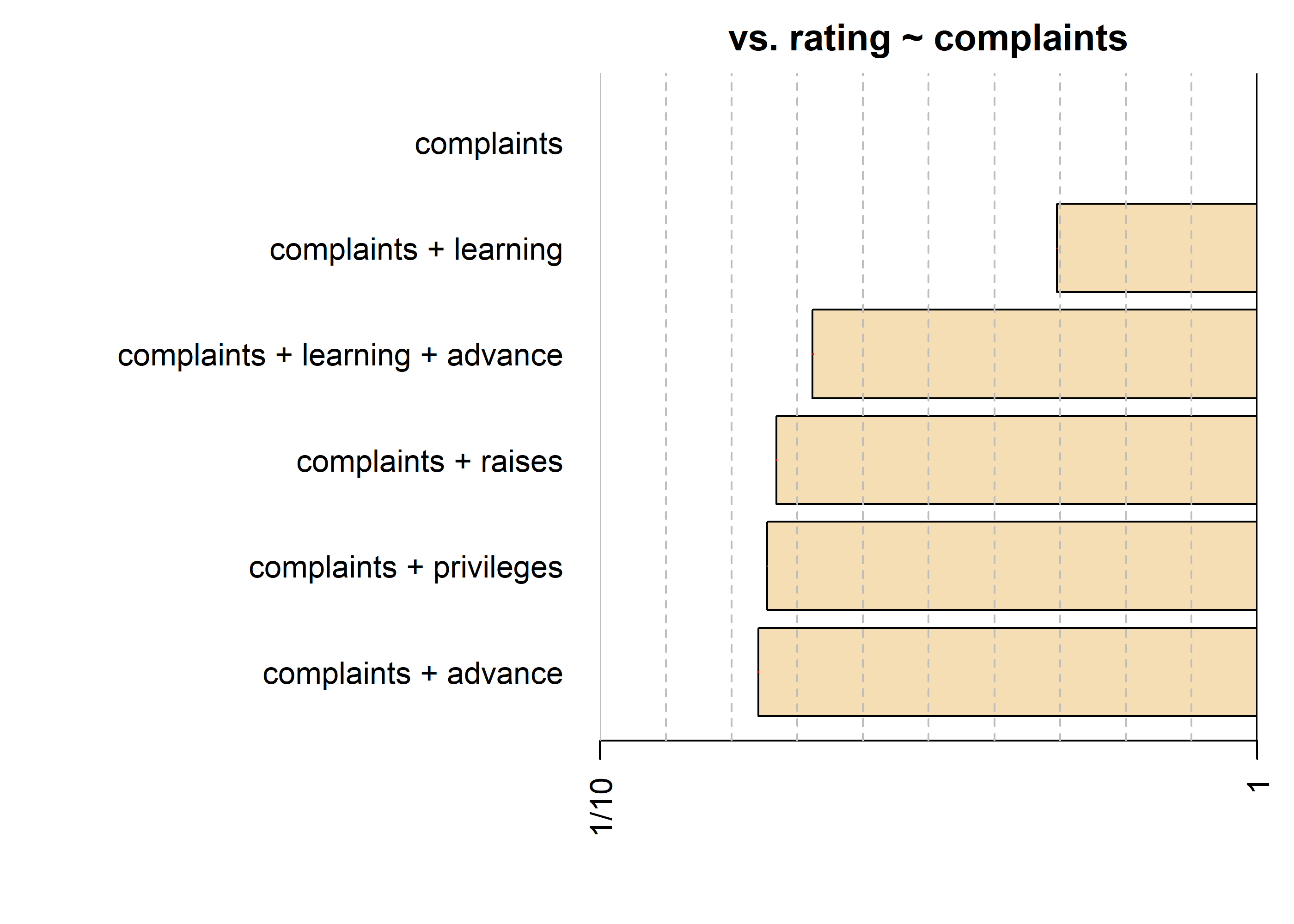The model preferred by Bayes factor is the complaints-only model, followed by the complaints + learning model, as might have been expected by the classical analysis.

We might also be interested in comparing the most complex model to all models that can be formed by removing a single covariate, or, similarly, comparing the intercept-only model to all models that can be formed by added a covariate. These comparisons can be done by setting the whichModels argument to 'top' and 'bottom', respectively. For example, for testing against the most complex model:

bf = regressionBF(rating ~ ., data = attitude, whichModels = "top")
## The seventh model is the most complex
bf
## Bayes factor top-down analysis
## --------------
## When effect is omitted from complaints + privileges + learning + raises + critical + advance , BF is...
##  Omit advance    : 1.73   ±0%
##  Omit critical   : 3.23   ±0%
##  Omit raises     : 3.13   ±0%
##  Omit learning   : 0.727  ±0%
##  Omit privileges : 2.92   ±0%
##  Omit complaints : 0.0231 ±0%
##
## Against denominator:
##   rating ~ complaints + privileges + learning + raises + critical + advance
## ---
## Bayes factor type: BFlinearModel, JZS
plot(bf)